The ever growing field – Data Science has numerous techniques and tools. It is not possible for anybody to remember all the formulas, operations and functions for each concept. That is why Cheat Sheet. So, I decided to write an article compiled of chosen right cheat sheet on the basis of content, clarity and comprehensiveness.

After filtering numerous sheets, I came up with collection of some cheat sheets on machine learning, python for data science and big data for Python Development. Following are the cheat sheets segregated according to the their topics on tools, techniques, libraries and language.

### Machine Learning Cheat Sheet

There are a handful of helpful flowcharts and tables of Machine Learning algorithms. I’ve included only the most comprehensive ones I’ve found.

#### 1. Microsoft Azure Machine Learning: Algorithm Cheat Sheet:

The best algorithms for your predictive analytics solution, developed by Microsoft Azure team.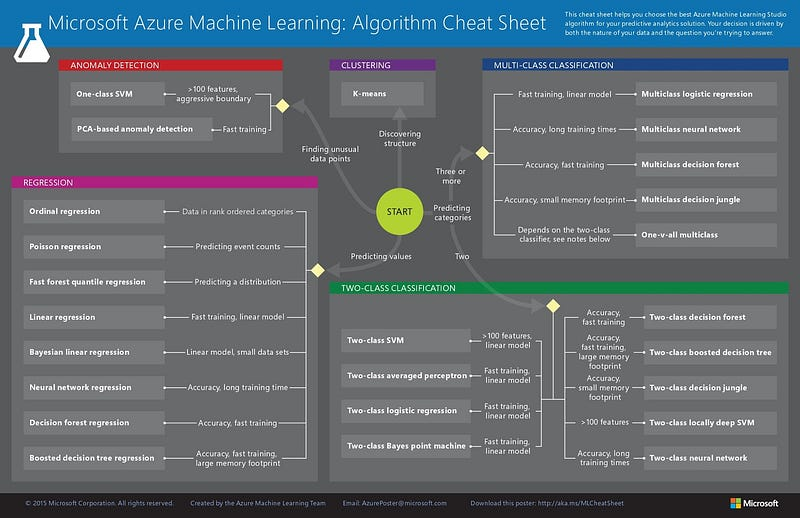#### 2. Python and R codes for common Machine Learning Algorithms:

Codes for Python & R for most commonly used machine learning algorithms, including Linear regression, logistics regression, decision tree, Naive Bayen, SVM, K-means,random forest and few others for Python development.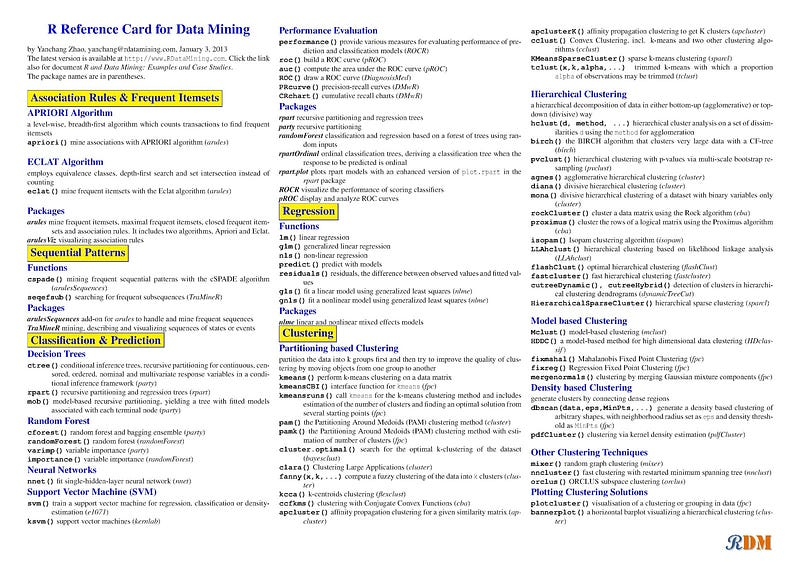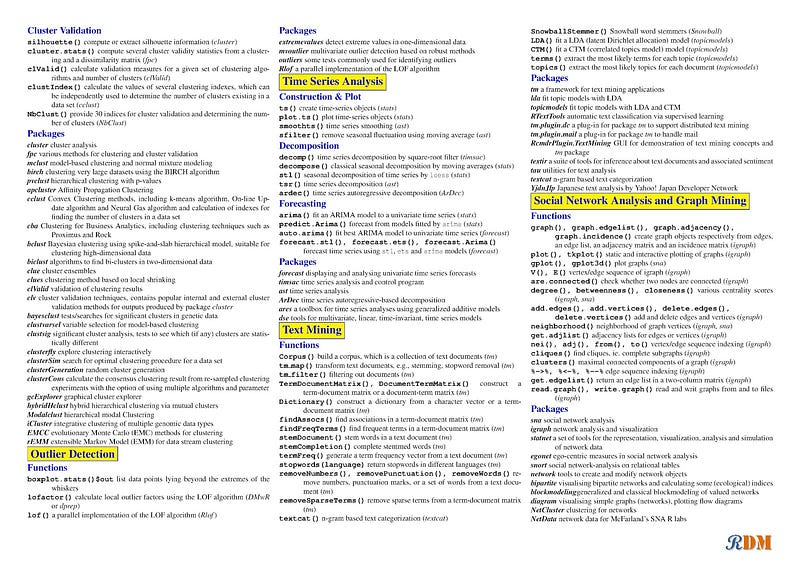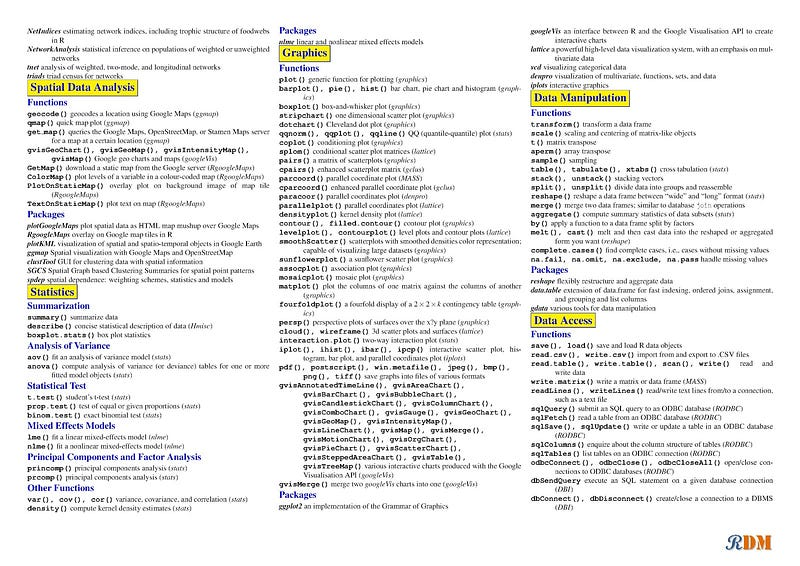####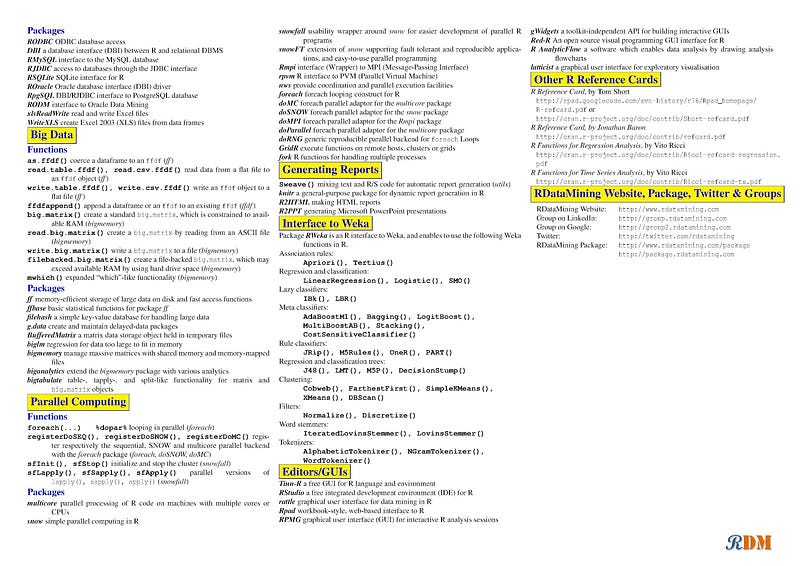3. Scikit Learn algorithm Cheat Sheet

The cheat sheet from the maker of scikit-learn, for people facing problem of choosing machine learning algorithm for different problems and data types. Have complete flow of solving a machine learning problem, with the help of this cheat sheet.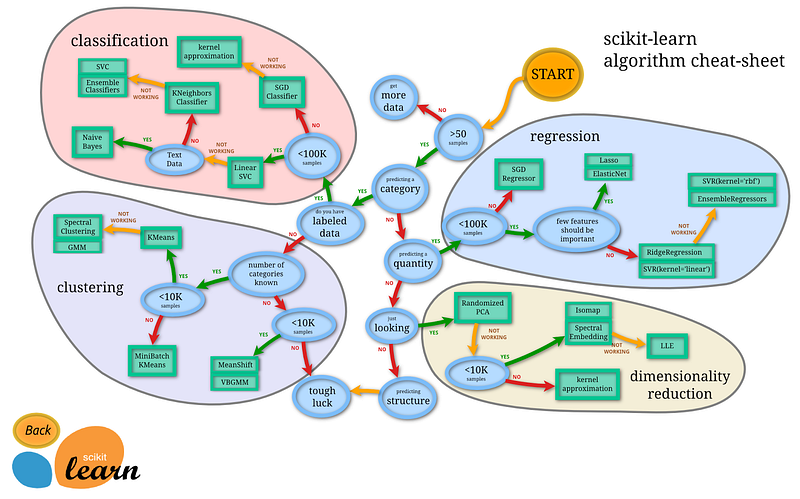### Python Development for Data Science Cheat Sheet

#### 1. Python for Data Science:

The cheat sheet for basics of Python development for data science, by Datacamp. This could be used for quick reference if you have just started working on Python. This sheet is list down to all important Python libraries, variable, data type functions, string operations, type conversion, lists and commonly used NumPy operations.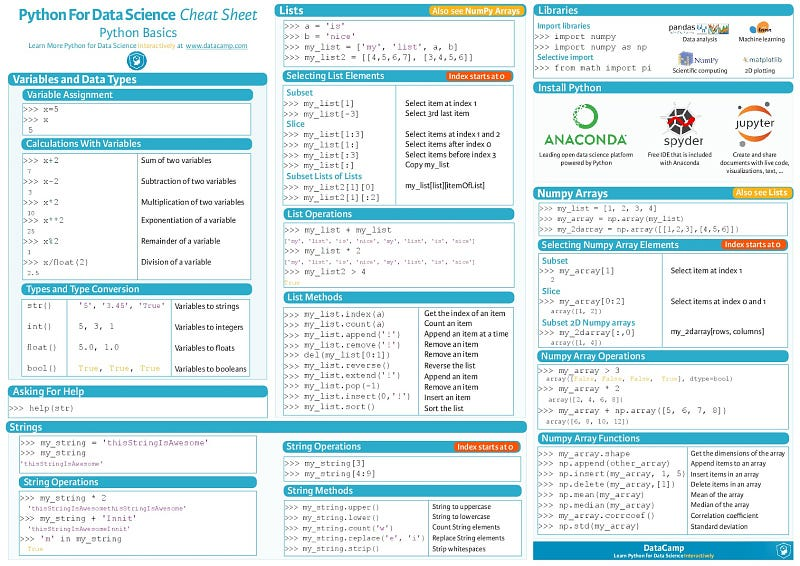#### 2. Python Development for Data Science NumPy:

NumPy – A core library for scientific computing in Python. Find cheat codes for creating NumPy arrays, performing mathematics operation on array, slicing, array manipulation, subsetting and indexing.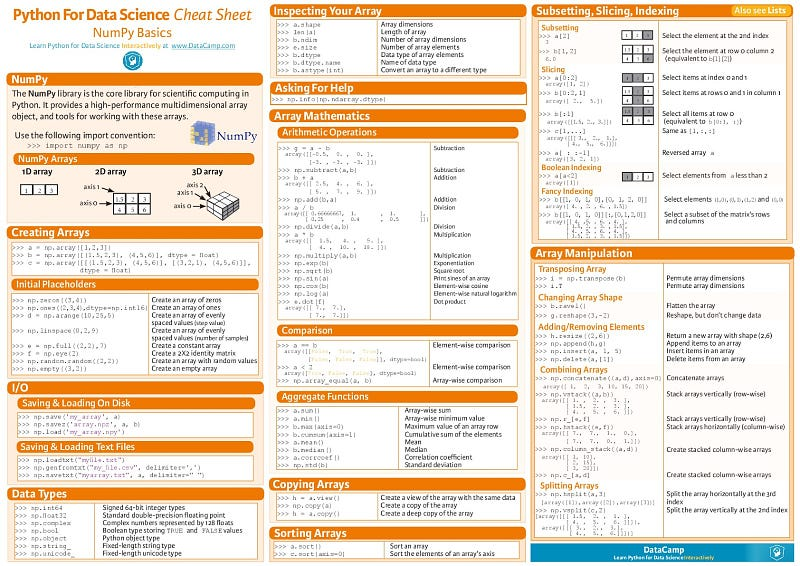#### 3.Python for Data Science Cheat Sheet Bokeh

An interactive visualization library in Python with large datasets by Datacamp, will get basic steps for plotting, renderers & visual customization, save plots & create statistical charts.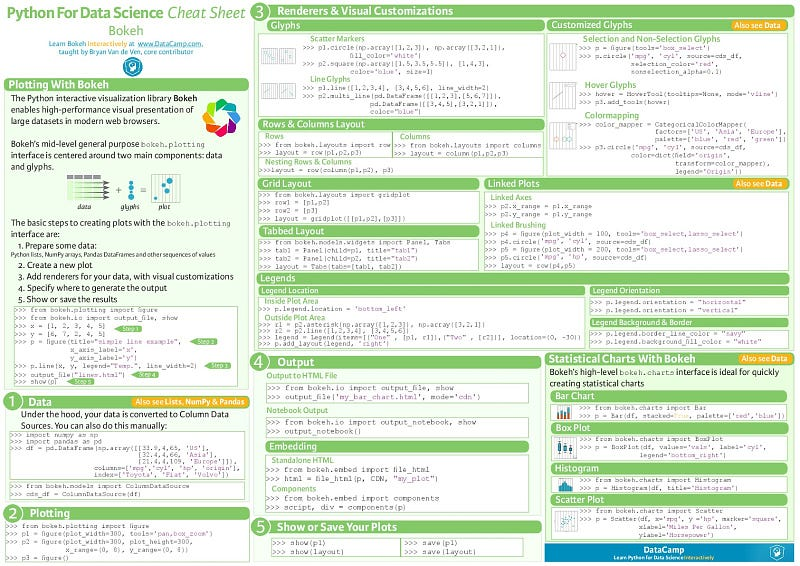I hope this article helps you. If you find any cheat sheet missing that should be added to the list, let me know in the comment section. I would like to know about them. Also, tell me what more cheat sheets you would like me to publish.

Want to work with us? We're hiring!